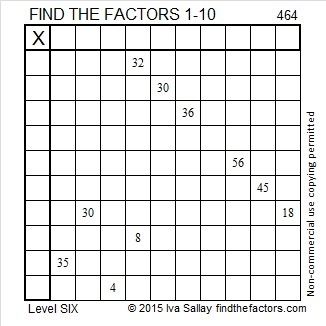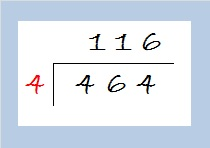# 464 and Level 6

464 is the hypotenuse of Pythagorean triple 320-336-464. Can you figure out what is the greatest common factor of those three numbers? Hint: it has to be an even factor of 320 because that is the smallest of those three even numbers.

The logic needed to begin this Level 6 puzzle shouldn’t be too difficult to discover.Print the puzzles or type the solution on this excel file:  10 Factors 2015-04-13

—————————————————————————————————

If I wanted to simplify √464, I would first notice that its last two digits, 64, are divisible by 4, so 464 also is divisible by 4. I would make a little cake like this:464 ÷ 4 = 116. Guess what? 116 is also divisible by 4 because 16 is divisible by 4. I would make another layer for my cake like this:29 is a prime number so my cake is finished. Now to simplify √464, I would just take the square root of everything on the outside of the cake and multiply them together.

√464 = (√4)(√4)(√29) = 4√29

• 464 is a composite number.
• Prime factorization: 464 = 2 x 2 x 2 x 2 x 29, which can be written 464 = (2^4) x 29
• The exponents in the prime factorization are 4 and 1. Adding one to each and multiplying we get (4 + 1)(1 + 1) = 5 x 2 = 10. Therefore 464 has exactly 10 factors.
• Factors of 464: 1, 2, 4, 8, 16, 29, 58, 116, 232, 464
• Factor pairs: 464 = 1 x 464, 2 x 232, 4 x 116, 8 x 58, or 16 x 29
• Taking the factor pair with the largest square number factor, we get √464 = (√16)(√29) = 4√29 ≈ 21.5407—————————————————————————————————## 2 thoughts on “464 and Level 6”

1.Paula Beardell Krieg

The biggest factor, is it a sweet number?

•ivasallay

Yes, it’s pretty sweet!

This site uses Akismet to reduce spam. Learn how your comment data is processed.